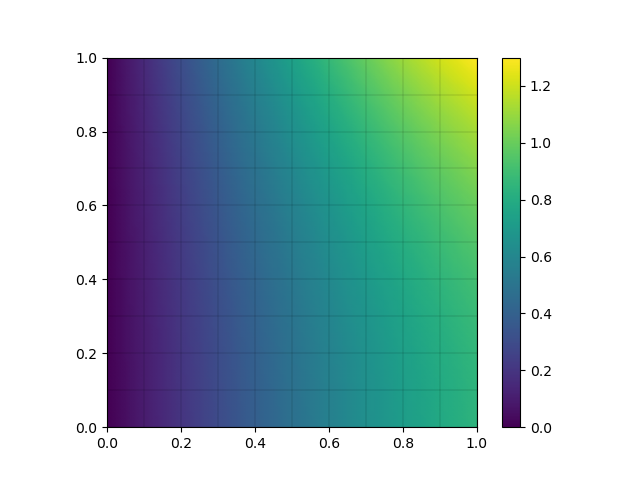By Evalf, and other Nutils contributors

officialLaplace

Solves Laplace's equation Δu = 0 on a unit square domain Ω with boundary Γ, subject to boundary conditions:

    u = 0                 Γ_left
∂_n u = 0                 Γ_bottom
∂_n u = cos(1) cosh(y)    Γ_right
u = cosh(1) sin(x)    Γ_top


This problem is constructed to contain all combinations of homogenous and heterogeneous, Dirichlet and Neumann type boundary conditions, as well as to have a known exact solution in uexact = sin(x) cosh(y).